Project tutorial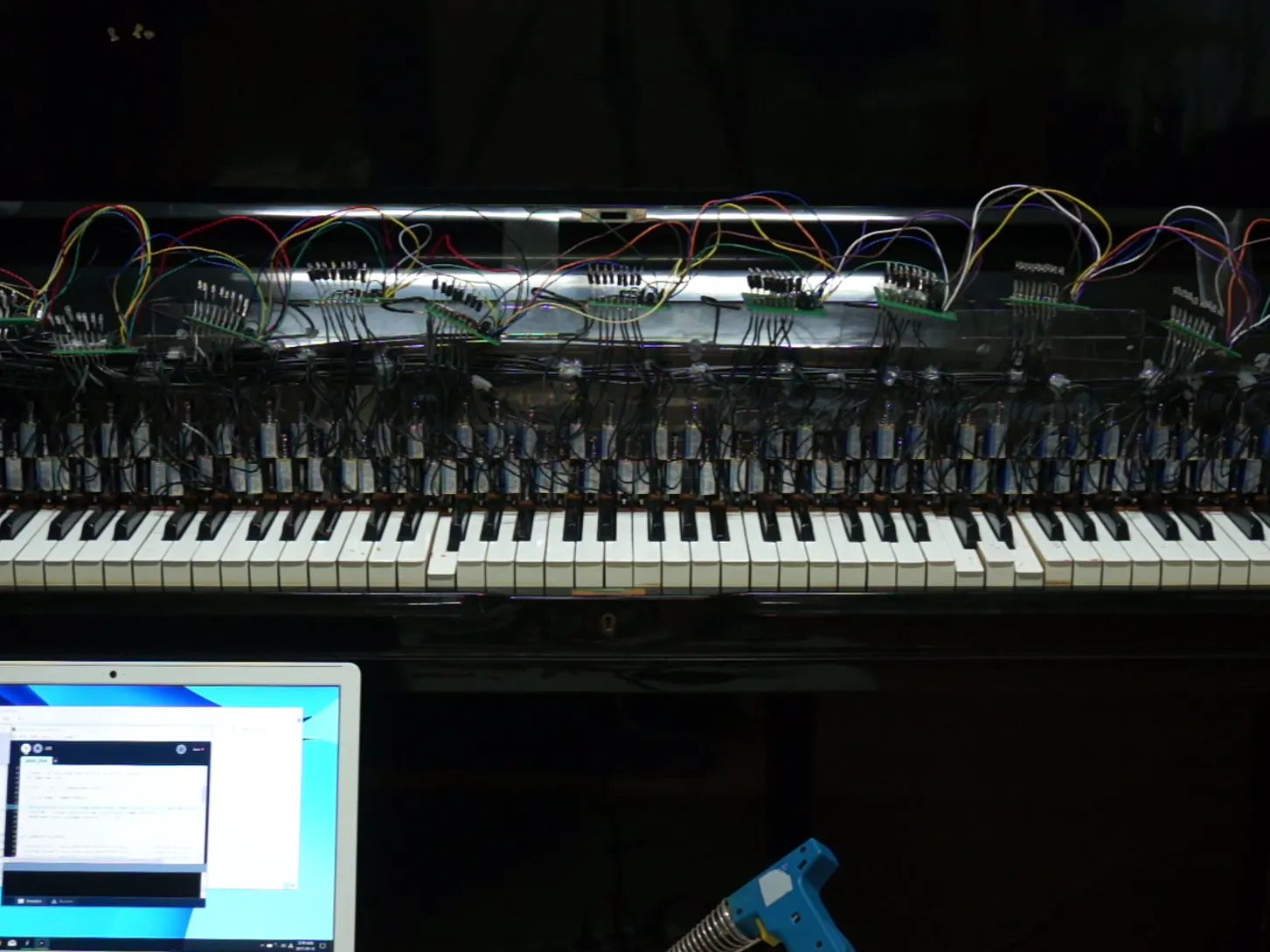Arduino Controlled Piano Robot: PiBot © GPL3+

Using one Arduino Uno board to control 88 solenoids to play piano.

• 12,568 views
• 49 respects

Components and supplies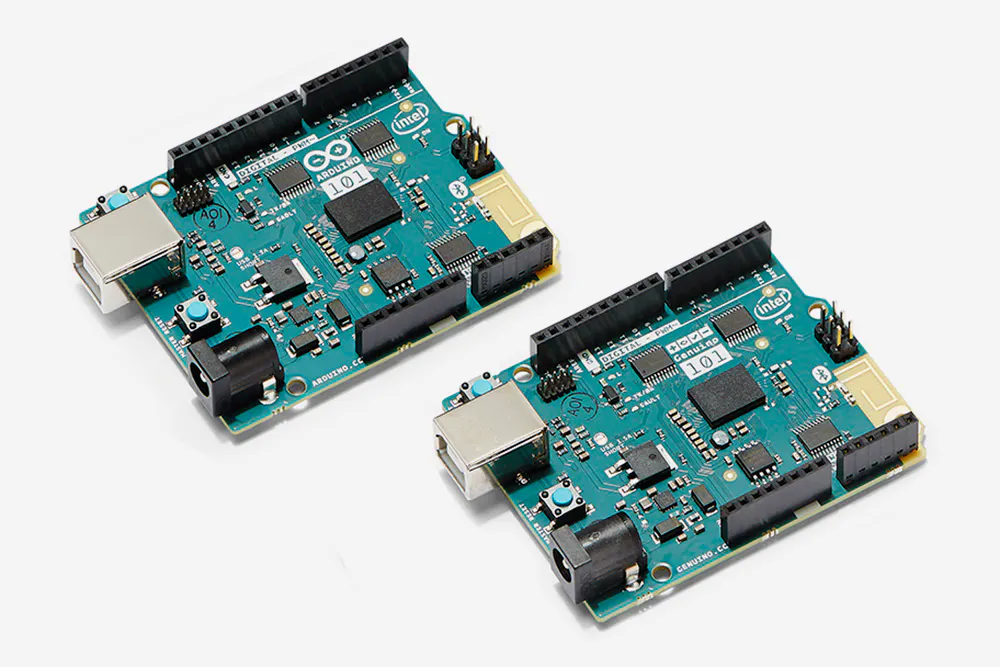Arduino 101 & Genuino 101
×1
 Texas Instruments 74HC595
×11
 IRFZ44N N-Channel Mosfet
×88
 12V Battery
×2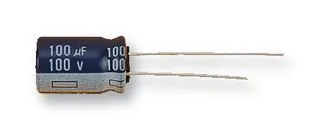Capacitor 100 µF
×11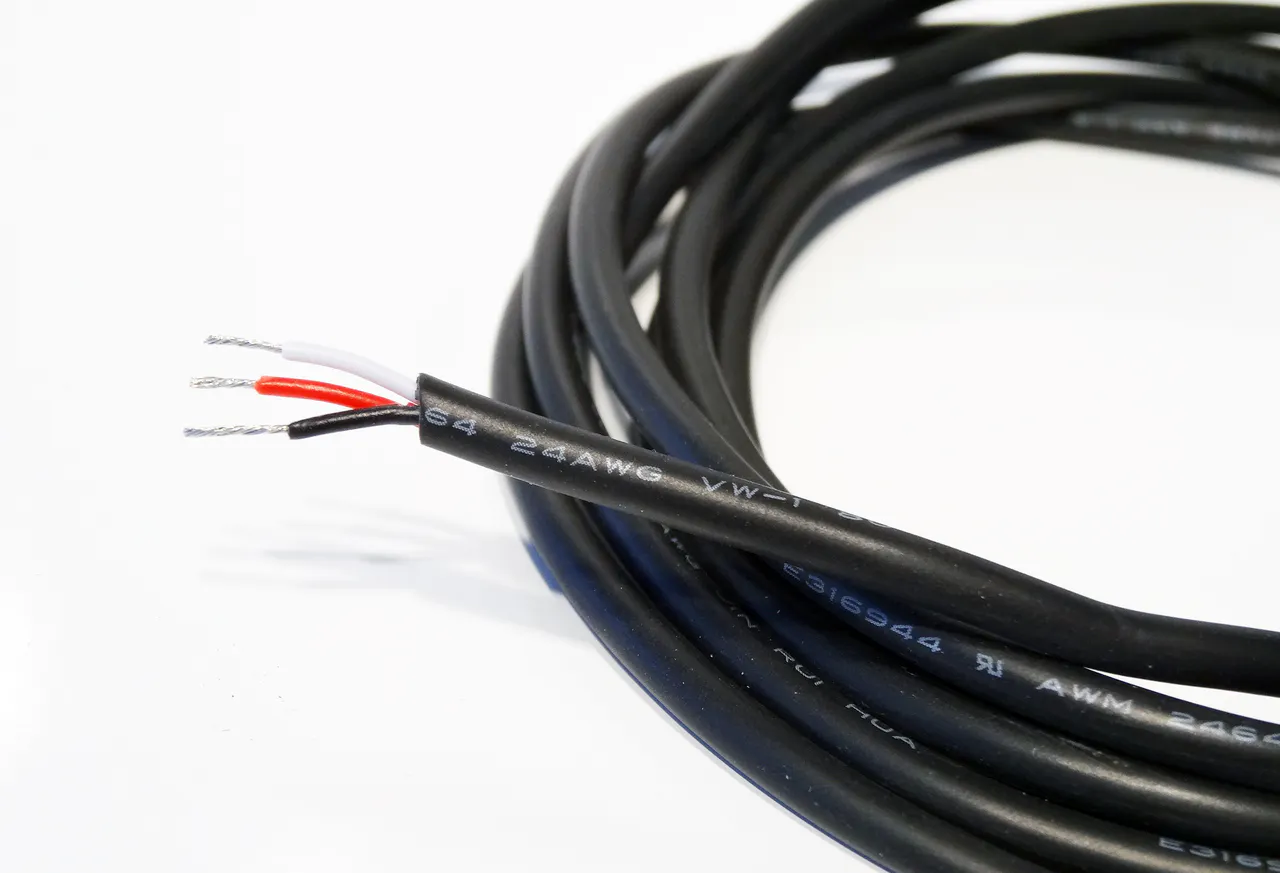OpenBuilds Wire Cable - By the Foot a lot of them.
×1Breadboard (generic) Or generic prototype board. Just about anything that will put things together.
×11
 8 legs IC socket Optional if you're confident that you won't burn ANY of these expensive 595
×11Resistor 10k ohm Not used for this project but better to have for each mosfet
×88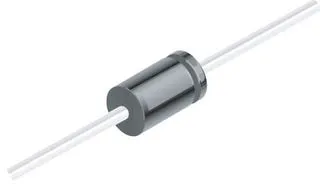1N4007 – High Voltage, High Current Rated Diode Not used for this project but better to have for each mosfet
×88

Necessary tools and machinesHot glue gun (generic)

Apps and online services

 Processing
 Python
 Binary MIDI file to text

How it begin:

Many years ago, Yamaha introduced automated piano. Young and innocent me saw that piano playing music behind glass window of an instrument shop.

Enough of small talks, there really isn't big reason why I started this project besides I just wanted to.

Overview:

A single board of Arduino Mega costs about \$40 and two will be required to control 88 solenoids. That's quite expensive. Instead, get a cheap Arduino Uno and 11 of shift-register. Shift Register is a method to control many outputs (usually LEDs) with small number of output pins. Essentially, it's one Arduino with 11 shift registers and control 88 Solenoids.

Since we're using Shift registers, a PC will send a set of bits to Arduino instead of MIDI com. MIDI file will be translated into set of bits before hand.

Hardware:

When I got the solenoids straight from China, I found out that these solenoids are not strong enough to push piano keys. Of course pushing piano keys from inner most spot takes more force but I thought it was the best method that doesn't wreck a piano. Eventually I pushed 24V through 12V solenoids to get enough power.

88 Solenoids consume a lot of power and because I can't go and buy an expensive PSU, I decided go with my dad's car battery. (Guess he won't be going anywhere now!)

With that out of the way, each one of shift registers and MOSFETs will go on a controller board.

595 on the right with a socket in case I burn it. (Which I did once.) Circuit Diagram is exactly same as example 2 from here. Replace LEDs with MOSFET gate. As you can see, there's no pull down resistor because extra resistors will bring the cost up and soldering them on that board will melt my fingers. On a bit more serious side, these MOSFETs will open at 5V and close under 4V or so. Confirmed it through countless hours of testing. (Not theoretically correct. Do not listen to me.)

Lastly, get a peice of plastic plate to glue solenoids on to. Using hot glue and plastic plate is a bad idea considering that it will get hot, but it's the best I can afford.

And then run one side of solenoid wires to the positive side of the battery.

Software:

The very first step is to get a midi file.

The second step is to get the midi into the text form. This can be done on this handy website: http://flashmusicgames.com/midi/mid2txt.php.

For the sake of simplicity, ignore time signature, tempo, and par. Tempo can be multiplied to the time later. Essentially you want a file like this:

Now, use this to create 11 sets of 8 bit data with time by running it through the Python code (attached).

They are ready to be sent to Arduino through processing COM.

See the attachment to figure out how processing sends these data and how Arduino handles them.

*Note: My coding habits are bad and it might be difficult to read these. Processing sends data from the right to left because Arduino pushes data to right on the physical piano.

Troubleshooting:

If a professional engineer were to see this post, he will think that this entire system will have many problems. And there are many problems.

Since the solenoids were hot glued to the plate, solenoids overheating and melting the hot glue was a big problem. The solution was to simply remove them and replace it with a double sided tape that can withstand up to 150C.

Code

Python Code for translationPython
Takes textified mifi file and translates it to 11 sets of binary for arduino to take it.
output_file = open("translated.txt", "w")
input_file = open("megalocania.txt")
work_data = raw_input_data.splitlines()
result = []

#output_file = open("result.txt", "w")

def main():

for a in work_data:
temp_time = time_finder(a)

if result == []:
result.append(str(temp_time) + ",")
if on_off_finder(a):
result[-1] += set_bit(True, note_finder(a))
elif not on_off_finder(a):
result[-1] += set_bit(True, note_finder(a))

elif time_finder_comm(result[-1]) == temp_time:
result[-1] = str(temp_time) + "," + set_bit_prev(on_off_finder(a), note_finder(a), -1)

elif time_finder_comm(result[-1]) != temp_time:
result.append(str(temp_time) + "," + set_bit_prev(on_off_finder(a), note_finder(a), -1))

for b in result:
output_file.write(b)
output_file.write("\n")

output_file.close()

def set_bit(On, note):
#Takes boolean for if it is on or not, and note number.
#Generates bit
if(note >= 21 and note <= 28 and On):
return str(2**(note - 21)) + ",0,0,0,0,0,0,0,0,0,0"
elif(note >= 29 and note <= 36 and On):
return "0," + str(2**(note - 29)) + ",0,0,0,0,0,0,0,0,0"
elif(note >= 37 and note <= 44 and On):
return "0,0," + str(2**(note - 37)) + ",0,0,0,0,0,0,0,0"
elif(note >= 45 and note <= 52 and On):
return "0,0,0," + str(2**(note - 45)) + ",0,0,0,0,0,0,0"
elif(note >= 53 and note <= 60 and On):
return "0,0,0,0," + str(2**(note - 53)) + ",0,0,0,0,0,0"
elif(note >= 61 and note <= 68 and On):
return "0,0,0,0,0," + str(2**(note - 61)) + ",0,0,0,0,0"
elif(note >= 69 and note <= 76 and On):
return "0,0,0,0,0,0," + str(2**(note - 69)) + ",0,0,0,0"
elif(note >= 77 and note <= 84 and On):
return "0,0,0,0,0,0,0," + str(2**(note - 77)) + ",0,0,0"
elif(note >= 85 and note <= 92 and On):
return "0,0,0,0,0,0,0,0," + str(2**(note - 85)) + ",0,0"
elif(note >= 93 and note <= 100 and On):
return "0,0,0,0,0,0,0,0,0," + str(2**(note - 93)) + ",0"
elif(note >= 101 and note <= 108 and On):
return "0,0,0,0,0,0,0,0,0,0," + str(2**(note - 101))
else:
return "0,0,0,0,0,0,0,0,0,0,0"

def set_bit_prev(On, note, index):
#Same as set_bit but previous aware
temp = result[index]
temp = temp[(temp.find(",") + 1):]

if(note >= 21 and note <= 28):
local_temp = temp[0:temp.find(",")]
if(On):
return str(int(local_temp) + (2**(note - 21))) + temp[temp.find(","):]
if(not On):
return str(int(local_temp) - (2**(note - 21))) + temp[temp.find(","):]

elif(note >= 29 and note <= 36):
local_temp = temp[(temp.find(",") + 1):indexTh(temp, ",", 2)]
if(On):
return temp[0:temp.find(",") + 1] + str(int(local_temp) + (2**(note - 29))) + temp[indexTh(temp, ",", 2):]
if(not On):
return temp[0:temp.find(",") + 1] + str(int(local_temp) - (2**(note - 29))) + temp[indexTh(temp, ",", 2):]

elif(note >= 37 and note <= 44):
local_temp = temp[(indexTh(temp, ",", 2) + 1):indexTh(temp, ",", 3)]
if(On):
return temp[0:indexTh(temp, ",", 2) + 1] + str(int(local_temp) + (2**(note - 37))) + temp[indexTh(temp, ",", 3):]
if(not On):
return temp[0:indexTh(temp, ",", 2) + 1] + str(int(local_temp) - (2**(note - 37))) + temp[indexTh(temp, ",", 3):]

elif(note >= 45 and note <= 52):
local_temp = temp[(indexTh(temp, ",", 3) + 1):indexTh(temp, ",", 4)]
if(On):
return temp[0:indexTh(temp, ",", 3) + 1] + str(int(local_temp) + (2**(note - 45))) + temp[indexTh(temp, ",", 4):]
if(not On):
return temp[0:indexTh(temp, ",", 3) + 1] + str(int(local_temp) - (2**(note - 45))) + temp[indexTh(temp, ",", 4):]

elif(note >= 53 and note <= 60):
local_temp = temp[(indexTh(temp, ",", 4) + 1):indexTh(temp, ",", 5)]
if(On):
return temp[0:indexTh(temp, ",", 4) + 1] + str(int(local_temp) + (2**(note - 53))) + temp[indexTh(temp, ",", 5):]
if(not On):
return temp[0:indexTh(temp, ",", 4) + 1] + str(int(local_temp) - (2**(note - 53))) + temp[indexTh(temp, ",", 5):]

elif(note >= 61 and note <= 68):
local_temp = temp[(indexTh(temp, ",", 5) + 1):indexTh(temp, ",", 6)]
if(On):
return temp[0:indexTh(temp, ",", 5) + 1] + str(int(local_temp) + (2**(note - 61))) + temp[indexTh(temp, ",", 6):]
if(not On):
return temp[0:indexTh(temp, ",", 5) + 1] + str(int(local_temp) - (2**(note - 61))) + temp[indexTh(temp, ",", 6):]

elif(note >= 69 and note <= 76):
local_temp = temp[(indexTh(temp, ",", 6) + 1):indexTh(temp, ",", 7)]
if(On):
return temp[0:indexTh(temp, ",", 6) + 1] + str(int(local_temp) + (2**(note - 69))) + temp[indexTh(temp, ",", 7):]
if(not On):
return temp[0:indexTh(temp, ",", 6) + 1] + str(int(local_temp) - (2**(note - 69))) + temp[indexTh(temp, ",", 7):]

elif(note >= 77 and note <= 84):
local_temp = temp[(indexTh(temp, ",", 7) + 1):indexTh(temp, ",", 8)]
if(On):
return temp[0:indexTh(temp, ",", 7) + 1] + str(int(local_temp) + (2**(note - 77))) + temp[indexTh(temp, ",", 8):]
if(not On):
return temp[0:indexTh(temp, ",", 7) + 1] + str(int(local_temp) - (2**(note - 77))) + temp[indexTh(temp, ",", 8):]

elif(note >= 85 and note <= 92):#error here
local_temp = temp[(indexTh(temp, ",", 8) + 1):indexTh(temp, ",", 9)]
if(On):
return temp[0:indexTh(temp, ",", 8) + 1] + str(int(local_temp) + (2**(note - 85))) + temp[indexTh(temp, ",", 9):]
if(not On):
return temp[0:indexTh(temp, ",", 8) + 1] + str(int(local_temp) - (2**(note - 85))) + temp[indexTh(temp, ",", 9):]

elif(note >= 93 and note <= 100):
local_temp = temp[(indexTh(temp, ",", 9) + 1):indexTh(temp, ",", 10)]
if(On):
return temp[0:indexTh(temp, ",", 9) + 1] + str(int(local_temp) + (2**(note - 93))) + temp[indexTh(temp, ",", 10):]
if(not On):
return temp[0:indexTh(temp, ",", 9) + 1] + str(int(local_temp) - (2**(note - 93))) + temp[indexTh(temp, ",", 10):]

elif(note >= 101 and note <= 108):
local_temp = temp[(indexTh(temp, ",", 10) + 1):]
if(On):
return temp[0:indexTh(temp, ",", 10) + 1] + str(int(local_temp) + (2**(note - 101)))
if(not On):
return temp[0:indexTh(temp, ",", 10) + 1] + str(int(local_temp) - (2**(note - 101)))

def indexTh(in_string, find_this, th):
#Takes String, string to find, and order to find string to find at that order
#returns index
order = 1
last_index = 0
while(True):
temp = in_string.find(find_this, last_index)
if(temp == -1):
return -1
if(order == th):
return temp
order += 1
last_index = temp + 1

def time_finder(in_string):
#Takes a string and finds time, returns it as an int
time_end = in_string.index(" ")
return int(in_string[0:time_end])

def time_finder_comm(in_string):
#Takes a string and finds time, returns it as an int comma
time_end = in_string.index(",")
return int(in_string[0:time_end])

def note_finder(in_string):
#Takes a string, looks for n=, returns n value as an int
num_start = in_string.index("n=") + 2
num_end = in_string.index("v=") - 1
return int(in_string[num_start:num_end])

def on_off_finder(in_string):
#takes a string, looks for On or Off, return true if On
start = in_string.index(" ") + 1
end = in_string.index("ch=") - 1
if in_string[start:end] == "On":
return True
elif in_string[start:end] == "Off":
return False

main()
Processing to send data to the arduinoProcessing
Reads the translated text file and sends it to the arduino.
Must mod tempo multiplier if tempo is different than 50000.
Reverses bytes because it shifts from left to right. (Text file assumes right to left)
import processing.serial.*;

Serial myPort;
String[] inputLines;

void setup()
{
myPort = new Serial(this, "COM3", 9600);
run();
}

void run()
{
//reads time and sends data bt line using data method
int lastTime = 0;

for(int i = 0; i < inputLines.length; i++)
{
String temp = inputLines[i];

//*5 is a tempo multiplier. increase the number if tempo is lower.
delay((Integer.parseInt(temp.substring(0, temp.indexOf(","))) - lastTime) * 5);
lastTime = Integer.parseInt(temp.substring(0, temp.indexOf(",")));
send(temp.substring(temp.indexOf(",") + 1));
}
}

void send(String data)
{
//String first = data.substring(indexOforder(data, ",", 1), (indexOforder(data, ",", 2) + 1));
//String second = data.substring(indexOforder(data, ",", 2), (indexOforder(data, ",", 3) + 1));
//String third = data.substring(indexOforder(data, ",", 3), (indexOforder(data, ",", 4) + 1));
//String forth = data.substring(indexOforder(data, ",", 4), (indexOforder(data, ",", 5) + 1));
//String fifth = data.substring(indexOforder(data, ",", 5), (indexOforder(data, ",", 6) + 1));
//String sixth = data.substring(indexOforder(data, ",", 6), (indexOforder(data, ",", 7) + 1));
//String seventh = data.substring(indexOforder(data, ",", 7), (indexOforder(data, ",", 8) + 1));
//String eighth = data.substring(indexOforder(data, ",", 8), (indexOforder(data, ",", 9) + 1));
//String ninth = data.substring(indexOforder(data, ",", 9), (indexOforder(data, ",", 10) + 1));
//String tenth = data.substring(indexOforder(data, ",", 10), (indexOforder(data, ",", 11) + 1));
//String eleventh = data.substring(indexOforder(data, ",", 11), (indexOforder(data, ",", 12) + 1));

//inverse declare
String eleventh = data.substring( 0 , indexOforder(data, ",", 1));
String tenth = data.substring((indexOforder(data, ",", 1) + 1), (indexOforder(data, ",", 2)));
String ninth = data.substring((indexOforder(data, ",", 2) + 1), (indexOforder(data, ",", 3)));
String eighth = data.substring((indexOforder(data, ",", 3) + 1), (indexOforder(data, ",", 4)));
String seventh = data.substring((indexOforder(data, ",", 4) + 1), (indexOforder(data, ",", 5)));
String sixth = data.substring((indexOforder(data, ",", 5) + 1), (indexOforder(data, ",", 6)));
String fifth = data.substring((indexOforder(data, ",", 6) + 1), (indexOforder(data, ",", 7)));
String forth = data.substring((indexOforder(data, ",", 7) + 1), (indexOforder(data, ",", 8)));
String third = data.substring((indexOforder(data, ",", 8) + 1), (indexOforder(data, ",", 9)));
String second = data.substring((indexOforder(data, ",", 9) + 1), (indexOforder(data, ",", 10)));
String first = data.substring(indexOforder(data, ",", 10) + 1);

myPort.write("888f");
myPort.write(first + "f");
myPort.write(second + "f");
myPort.write(third + "f");
myPort.write(forth + "f");
myPort.write(fifth + "f");
myPort.write(sixth + "f");
myPort.write(seventh + "f");
myPort.write(eighth + "f");
myPort.write(ninth + "f");
myPort.write(tenth + "f");
myPort.write(eleventh + "f");
myPort.write("999f");
}

int indexOforder(String data, String find, int order)
{
int currentOrder = 0;
int lastLocation = 0;

while(currentOrder < order)
{
lastLocation = data.indexOf(find, (lastLocation + 1));
currentOrder += 1;
}

return lastLocation;
}
Arduino codeArduino
Simple code for arduino. Takes inputs from Serial. 888 and 999 are reserved for shift register open and close command.

Schematics

I'm sorry for un-professional drawing. This is the whole concept. There's no difference between Arduino -ShiftOut document's diagram except for the mosfet. I recommend looking at that too.4-Wheel Robot Made With Arduino Controlled Using Dabble

Project tutorial by STEMpedia

• 6,781 views
• 25 respects

2WD Voice Controlled Robot with Arduino and BitVoicer Server

Project tutorial by Marcio Tamagushi

• 14,126 views
• 45 respects

Robot Arm Controlled Through Ethernet

Project tutorial by Balázs Simon

• 8,575 views
• 43 respects

Robot Controlled using HTML5/JavaScript and BeagleBone Green

Project tutorial by Dr. Charif Mahmoudi

• 8,506 views
• 35 respects

• 42,704 views